# Types of Chemical Reactions Writing Chemical Reactions Types

• Slides: 31Types of Chemical Reactions Writing Chemical ReactionsTypes of Reactions n Many chemical reactions have defining characteristics which allow them to be classified as to type.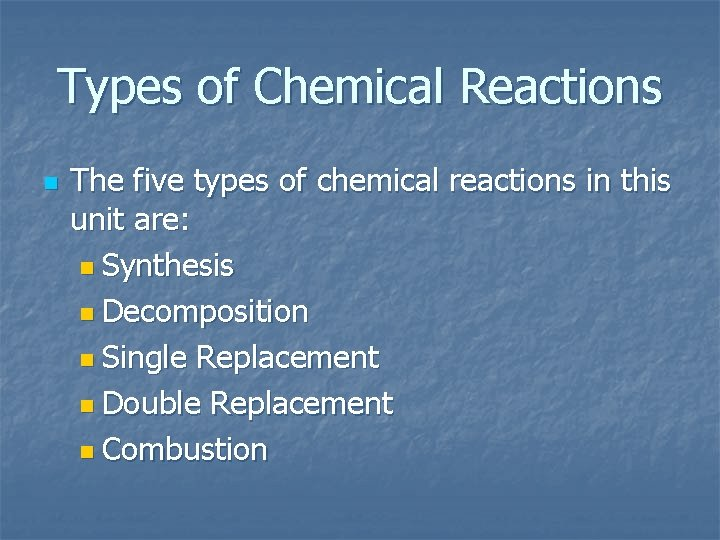Types of Chemical Reactions n The five types of chemical reactions in this unit are: n Synthesis n Decomposition n Single Replacement n Double Replacement n Combustion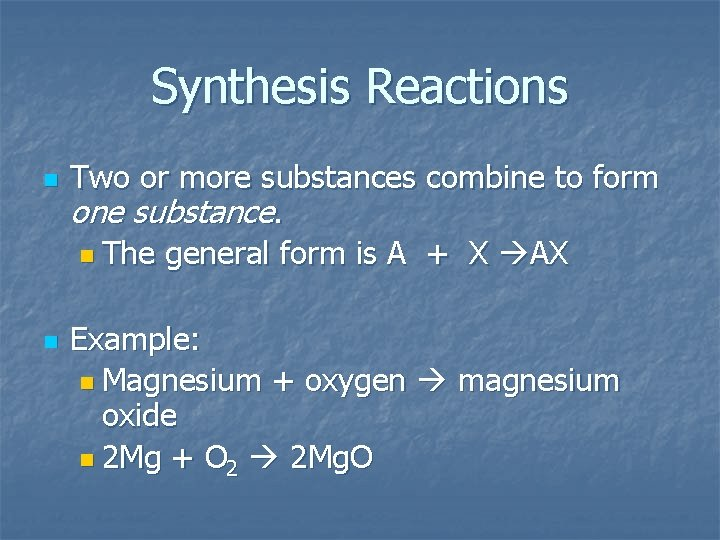Synthesis Reactions n n Two or more substances combine to form one substance. n The general form is A + X AX Example: n Magnesium + oxygen magnesium oxide n 2 Mg + O 2 2 Mg. O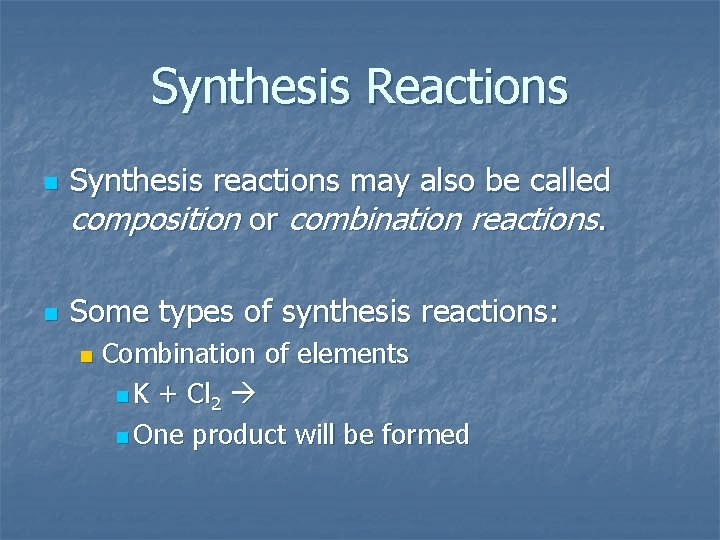Synthesis Reactions n n Synthesis reactions may also be called composition or combination reactions. Some types of synthesis reactions: n Combination of elements n K + Cl 2 n One product will be formed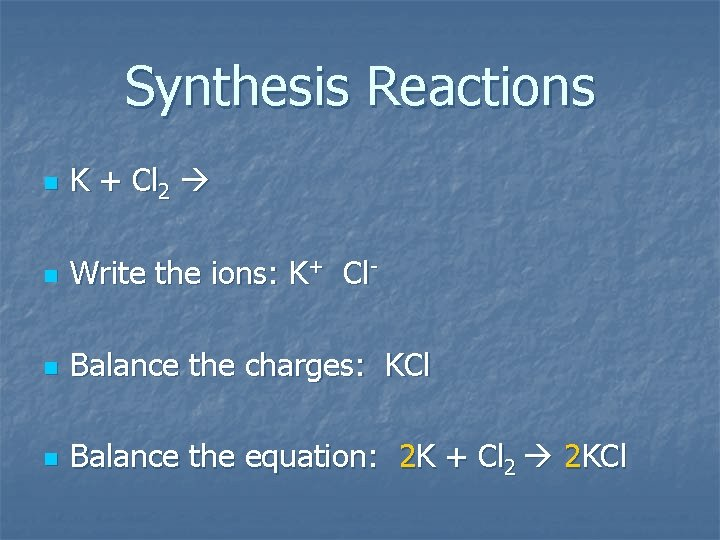Synthesis Reactions n K + Cl 2 n Write the ions: K+ Cl- n Balance the charges: KCl n Balance the equation: 2 K + Cl 2 2 KClSynthesis Reactions n Some types of synthesis reactions: n Oxide + water n Nonmetal oxide + water acid n SO 2 + H 2 O H 2 SO 3 n Metal oxide + water base n Ba. O + H 2 O Ba(OH)2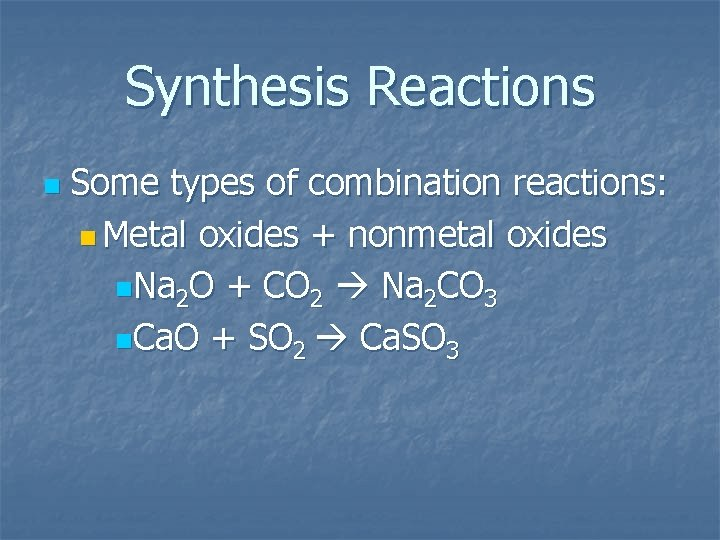Synthesis Reactions n Some types of combination reactions: n Metal oxides + nonmetal oxides n. Na 2 O + CO 2 Na 2 CO 3 n. Ca. O + SO 2 Ca. SO 3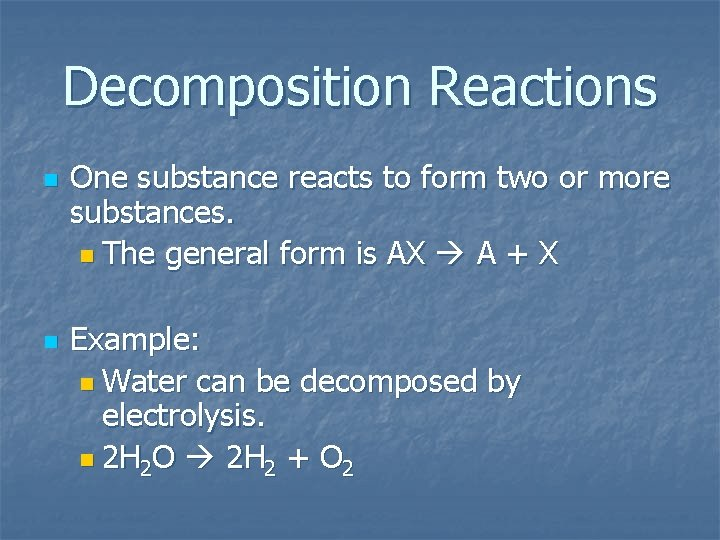Decomposition Reactions n n One substance reacts to form two or more substances. n The general form is AX A + X Example: n Water can be decomposed by electrolysis. n 2 H 2 O 2 H 2 + O 2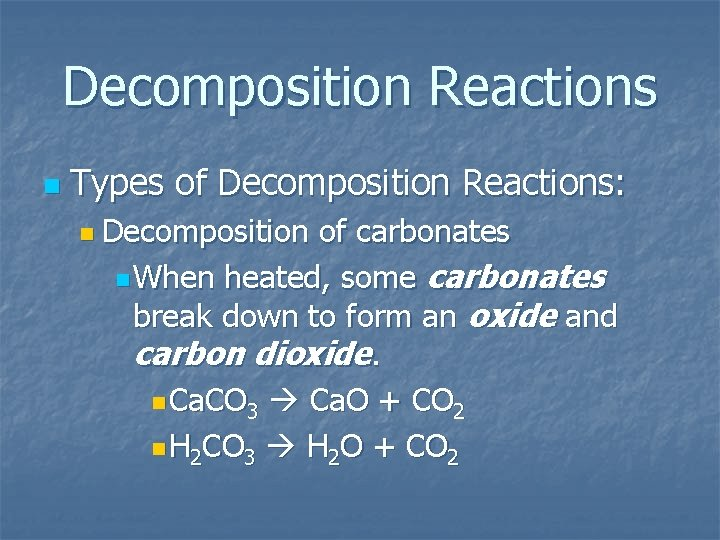Decomposition Reactions n Types of Decomposition Reactions: n Decomposition of carbonates n When heated, some carbonates break down to form an oxide and carbon dioxide. n Ca. CO 3 Ca. O + CO 2 n H 2 CO 3 H 2 O + CO 2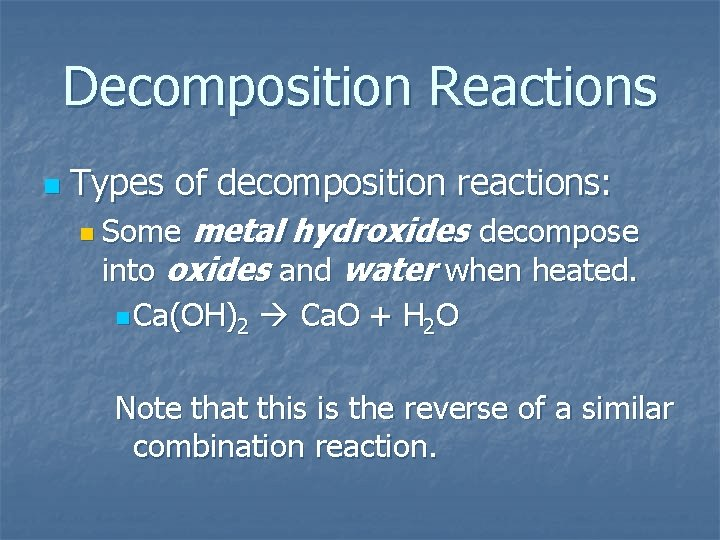Decomposition Reactions n Types of decomposition reactions: metal hydroxides decompose into oxides and water when heated. n Some n Ca(OH)2 Ca. O + H 2 O Note that this is the reverse of a similar combination reaction.Decomposition Reactions n Types of decomposition reactions: n Metal chlorates decompose into chlorides and oxygen when heated. n 2 KCl. O 3 2 KCl + 3 O 2 n Zn(Cl. O 3)2 Zn. Cl 2 + 3 O 2 n Some of these reactions are used in explosives.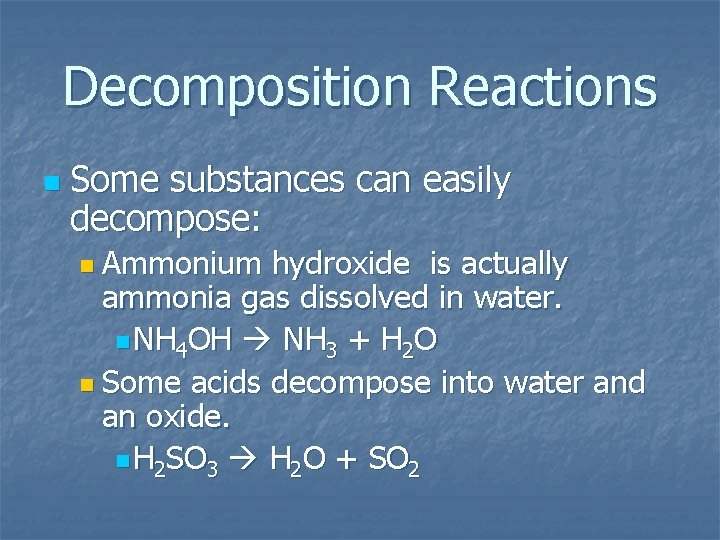Decomposition Reactions n Some substances can easily decompose: n Ammonium hydroxide is actually ammonia gas dissolved in water. n NH 4 OH NH 3 + H 2 O n Some acids decompose into water and an oxide. n H 2 SO 3 H 2 O + SO 2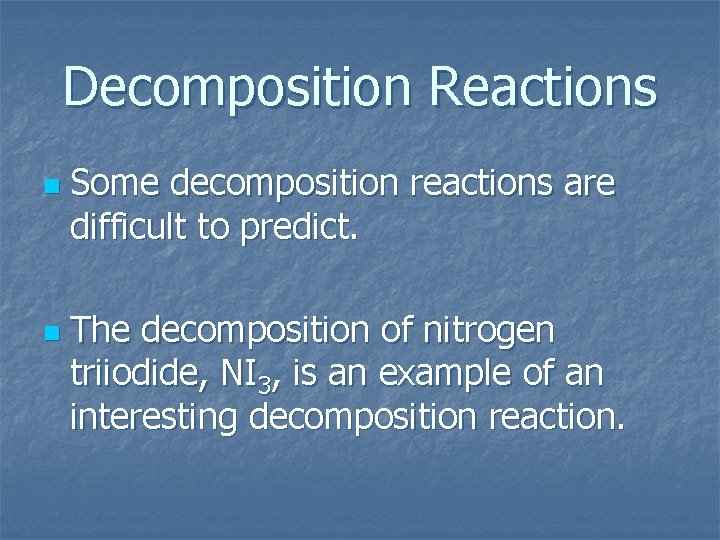Decomposition Reactions n n Some decomposition reactions are difficult to predict. The decomposition of nitrogen triiodide, NI 3, is an example of an interesting decomposition reaction.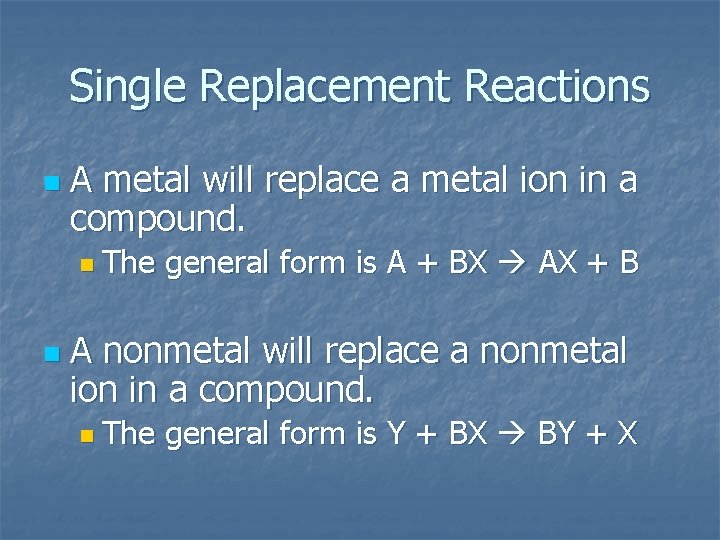Single Replacement Reactions n A metal will replace a metal ion in a compound. n The n general form is A + BX AX + B A nonmetal will replace a nonmetal ion in a compound. n The general form is Y + BX BY + XSingle Replacement Reactions n Examples: n Ni + Ag. NO 3 n Nickel replaces the metallic ion Ag+. n The silver becomes free silver and the nickel becomes the nickel(II) ion. n Ni + Ag. NO 3 Ag + Ni(NO 3)2 n Balance the equation: n Ni + 2 Ag. NO 3 2 Ag + Ni(NO 3)Single Replacement Reactions n n n Not all single replacement reactions that can be written actually happen. The metal must be more active than the metal ion. Aluminum is more active than iron in Al + Fe 2 O 3 in the following reaction:Thermite Reaction Al + Fe 2 O 3 n Aluminum will replace iron(III) as was seen in the video. n Iron(III) becomes Fe and aluminum metal becomes Al 3+. n 2 Al + Fe 2 O 3 2 Fe + Al 2 O 3 nSingle Replacement Reactions n An active nonmetal can replace a less active nonmetal. n The halogen (F 2, Cl 2, Br 2, I 2) reactions are good examples. n F 2 is the most active and I 2 is the least. n. Cl 2 +2 Na. I 2 Na. Cl + I 2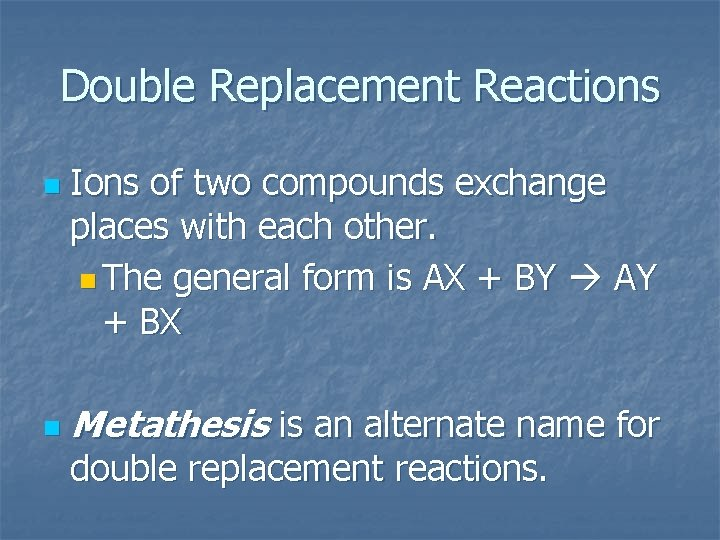Double Replacement Reactions n n Ions of two compounds exchange places with each other. n The general form is AX + BY AY + BX Metathesis is an alternate name for double replacement reactions.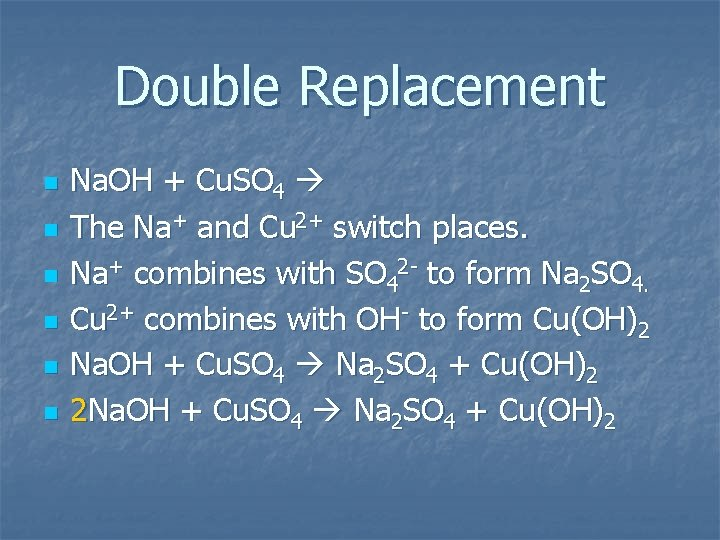Double Replacement n n n Na. OH + Cu. SO 4 The Na+ and Cu 2+ switch places. Na+ combines with SO 42 - to form Na 2 SO 4. Cu 2+ combines with OH- to form Cu(OH)2 Na. OH + Cu. SO 4 Na 2 SO 4 + Cu(OH)2 2 Na. OH + Cu. SO 4 Na 2 SO 4 + Cu(OH)2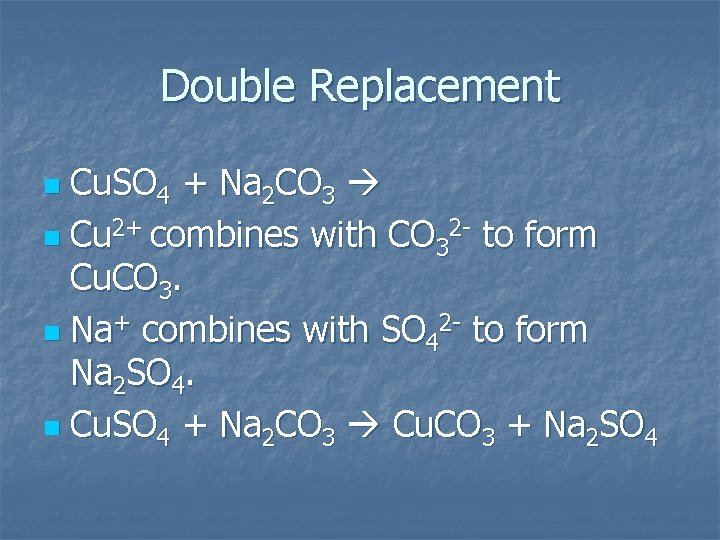Double Replacement Cu. SO 4 + Na 2 CO 3 n Cu 2+ combines with CO 32 - to form Cu. CO 3. n Na+ combines with SO 42 - to form Na 2 SO 4. n Cu. SO 4 + Na 2 CO 3 Cu. CO 3 + Na 2 SO 4 nDouble Replacement Na 2 CO 3 + HCl n Notice that gas bubbles were produced rather than a precipitate. n What was the gas? n Write the double replacement reaction first. nDouble Replacement Na 2 CO 3 + HCl n Na+ combines with Cl- to form Na. Cl. n H+ combines with CO 32 - to form H 2 CO 3. n Na 2 CO 3 + 2 HCl 2 Na. Cl + H 2 CO 3 n H 2 CO 3 breaks up into H 2 O and CO 2. nDouble Replacement The gas formed was carbon dioxide. n The final balanced reaction is: Na 2 CO 3 + HCl Na. Cl + H 2 O + CO 2. n Balance the equation. n Na 2 CO 3 + 2 HCl 2 Na. Cl + H 2 O + CO 2 n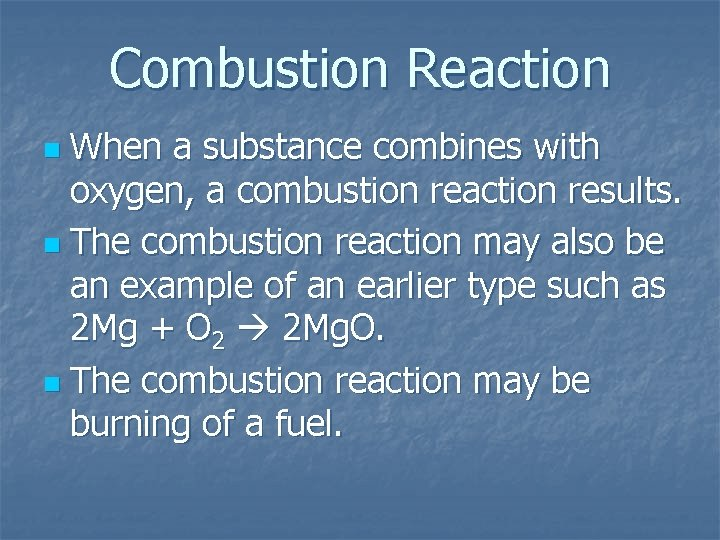Combustion Reaction When a substance combines with oxygen, a combustion reaction results. n The combustion reaction may also be an example of an earlier type such as 2 Mg + O 2 2 Mg. O. n The combustion reaction may be burning of a fuel. nCombustion Reaction Methane, CH 4, is natural gas. n When hydrocarbon compounds are burned in oxygen, the products are water and carbon dioxide. n CH 4 + O 2 CO 2 + H 2 O n CH 4 + 2 O 2 CO 2 + 2 H 2 O n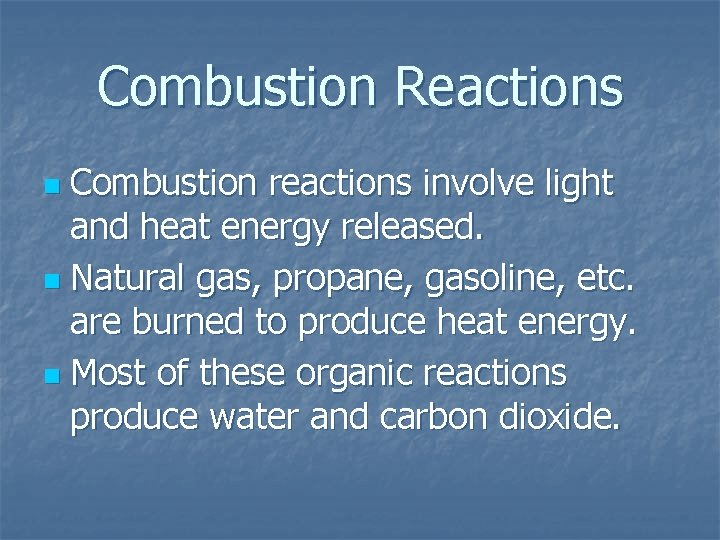Combustion Reactions Combustion reactions involve light and heat energy released. n Natural gas, propane, gasoline, etc. are burned to produce heat energy. n Most of these organic reactions produce water and carbon dioxide. n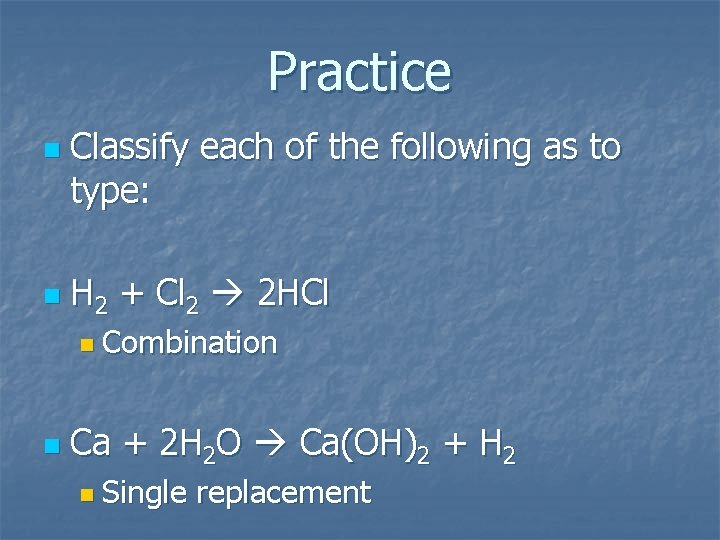Practice n n Classify each of the following as to type: H 2 + Cl 2 2 HCl n Combination n Ca + 2 H 2 O Ca(OH)2 + H 2 n Single replacement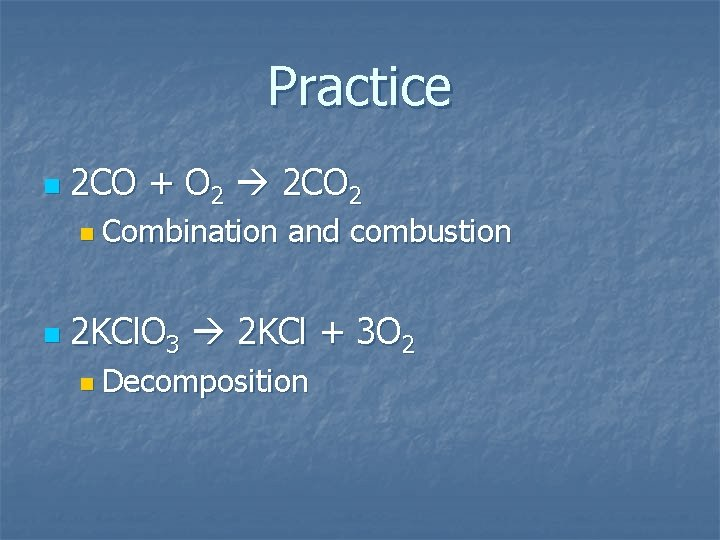Practice n 2 CO + O 2 2 CO 2 n Combination n and combustion 2 KCl. O 3 2 KCl + 3 O 2 n DecompositionPractice n Fe. S + 2 HCl Fe. Cl 2 + H 2 S n Double n replacement Zn + HCl ? n Single replacement n Zn + 2 HCl Zn. Cl 2 + H 2